# MCQs on Electrical Machines

##### Page 85 of 114. Go to page 1 2 3 4 5 6 7 8 9 10 11 12 13 14 15 16 17 18 19 20 21 22 23 24 25 26 27 28 29 30 31 32 33 34 35 36 37 38 39 40 41 42 43 44 45 46 47 48 49 50 51 52 53 54 55 56 57 58 59 60 61 62 63 64 65 66 67 68 69 70 71 72 73 74 75 76 77 78 79 80 81 82 83 84 85 86 87 88 89 90 91 92 93 94 95 96 97 98 99 100 101 102 103 104 105 106 107 108 109 110 111 112 113 114
01․ Torque angle of a synchorous machine operating from a constant voltage bus is usually defined as the space angle between
Stator mmf wave and resultant mmf wave.
Rotor mmf wave and resultant flux density wave.
Stator mmf wave and resultant flux density.
Rotor mmf wave and stator mmf wave.

As shown in the figure, V is applied voltage to stator and Eb is induced emf in the rotor, ± is the angle between two and is called load (or) torque angle because it depends on the load. It will be more if the load is more . Also, if ± is more than a limited value, then the loss of synchronism in the machine will occur.

02․ The result of slip test on a 3-phase, 20 KVA, 400 V, 50 Hz connected salient pole alternator is given below- Phase value: Vmax = 100 V, Vmin = 96 V, Imax = 10 A and I min = 7 A. The direct and quadranature axis reactances are respectively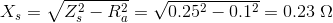$X_d = rac{V_{max}}{I_{min}} = rac{100}{7} = 14.25 \; \Omega$. $X_q = rac{V_{min}}{I_{max}} = rac{96}{10} = 9.6 \; \Omega;$

03․ If the field current is reduced to zero the maximum power developed by alternator is
1.644 KW.
2.73 KW.
5.46 KW.
0.9103 KW.

Maximum developed by alternator is given as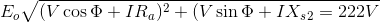[math] P_{max} = rac{V_t^2}{2} \left [ rac{1}{X_q} - rac{1}{X_d} ight].

04․ If dimension of all the parts of a synchorous generator and the number of field and armature turns are double, then generated voltage will change by a factor of
1.
2.
4.
8.

Phase emf induced = 4.44 WfNphφ, where symbol having usual meaning. On doubling diameter and length of the machine, the pole face area becomes four times the original area, air gap length doubles, thus, magnetic flux path reluctance becomes half of the original. Since field MMF has also been doubled, the flux φ shall be four times the original value. Armature turns also have been developed. Hence, generated voltage will change by a factor of 8.

05․ Find the synchronous reactance of alternators in which a given field current of 200 A on short circuit and a generated emf of 50 V on open circuit. Armature resistance is 0.1 ohm
0.23Ω.
0.25Ω.
0.5Ω.
1Ω.

Zs = 50/200 = 0.25 Ω.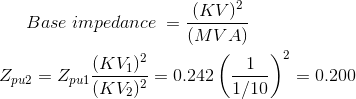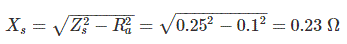06․ For the above problem, find the induced voltage of an alternator of it is to deliver a load of 100 A at power factor of 0.8 lagging with terminal voltage of 200 V.
220 V.
222 V.
225 V.
230 V.

IRa = 100 X 0.1 = 10 Volts IXs = 100 X 0.23 = 23 Volts. cosθ = 0.8, sinθ = 0.6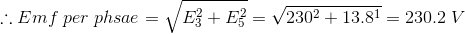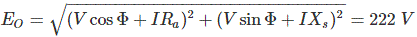07․ Voltage across the open circuited field termninals of a synchorous machine under slip test is
A modulated supply frequency AC voltage with slip frequency envelop.
AC of supply frequency.
DC.
DC of slip frequency.

When the driving power from the prime mover driving the alternator is lost, but alternator remain connected to the supply network and the field supply also remains, the alternator will behaves as a synchorous motor and will also continue to rotate the same direction.

08․ Two single-phase alternators operating in parallel have induced EMFs on open circuit of 230∠0° and 230∠10° volts and respective reactance of j2 Ω j3 Ω calculate the terminal voltage
150 V.
200 V.
223.V.
300 V.

E1 = 230∠0° Volts. E2 = 230∠10° Volts Z1 = j2 Ω Z2 = j3 Ω Z = 6 Ω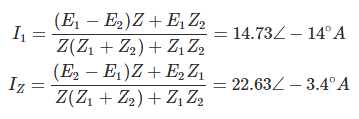Hence I = I1 + I2 = 37.2 ∠ -7.3° ∴ Terminal voltage, V = IZ = 223.2∠-7.3° Volts.

09․ In above questions, find the power delivered by each of the alternators to a load of impedance 6Ω(resistive)
100 W, 2000 W.
3190 W, 5040 W.
1000 W, 5040 W.
6000 W, 7000 W.

V = 223.2 ∠-7.3° Volts. I1 = 14.73∠-14° I2 = 22.63∠-3.4° P1 = VI1cosθ1 P2 = VI2cosθ2

10․ Two 3-phase AC generators are such that one has twice the linear dimensions of the other. The field windings of each are excited to give identical sinusoidal air-gap flux density waveform. Both have the same number of stator slots and identical winding patterns. The conductor/slot in a big generator is K times that of the smaller one. The value of K to get equal no load voltage at same frequency is
1/4.
1/2.
4.
8.

The area gap flux density waveform as a function of special angle¸ being the same, the bigger machine will give flux per pole = S2X θ. Where θ is flux per pole in the smaller machine. Therefore, to get equal no-load voltage, the number of series turns in the bigger machine should be half of that in the smaller one.

<<<8384858687>>>